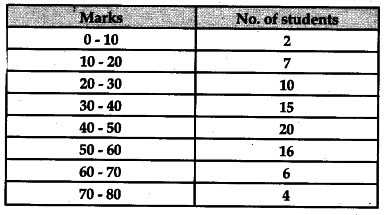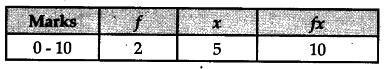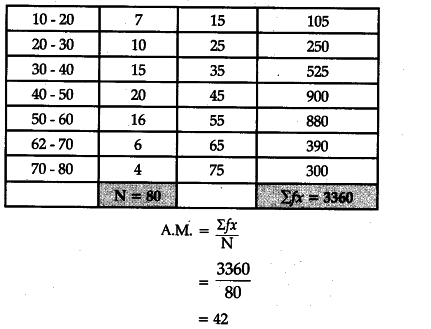# Calculate Arithmetic Mean from the following data:

Calculate Arithmetic Mean from the following data: